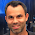Don't forget to also checkout my second blog containing articles to all other related ICT topics!!

Friday, June 1, 2012

Python: Creating dictionary of adjacent elements of circular iterable

In many problems you will need to deal with circular datastructures which are most of the time presented in a simple iterable. If you need to quickly find for a specific element what the left and right adjacent elements are, you can use this generic function presented below.
```#we got a list of persons sitting next to each other in a circle.
#This means Robby is sitting next to Alice (left) and Valerie (right) for below example.
#We want to create a quick lookup dict for who sits next to each other

def getCircularLookupDictionary(iter):
#generic function which returns a dict of items with format (key, (leftadjacent, rightadjacent))
l = len(iter)
"""
return dict(
(iter[i], (iter[l - 1], iter[i+1])  ) if i == 0 else
(iter[i], (iter[i - 1], iter)    ) if i == l -1 else
(iter[i], (iter[i - 1], iter[i+1])  ) for i in range(l)
)
"""
#nice oneliner provided by my colleague Ivan Lagunov !!
return dict((iter[i], (iter[i - 1], iter[i + 1 - l])) for i in range(l))

circle = ['Robby', 'Valerie', 'Lindsey', 'Audrey', 'Alice']
neighbours = getCircularLookupDictionary(circle)

print neighbours['Robby']
print neighbours['Lindsey']
print neighbours['Alice']

assert neighbours['Robby'] == ('Alice', 'Valerie')
assert neighbours['Lindsey'] == ('Valerie', 'Audrey')
assert neighbours['Alice'] == ('Audrey', 'Robby')
```

```('Alice', 'Valerie')
('Valerie', 'Audrey')
('Audrey', 'Robby')
```

We can even make it more generic if we want to specify what keys and values to use from the iterables.
```#we got a list of persons sitting next to each other in a circle.
#This means Robby is sitting next to Alice (left) and Valerie (right) for below example.
#We want to create a quick lookup dict for who sits next to each other

def getCircularLookupDictionary(iter, keyf, valuef):
#generic function which returns a dict of items with format (key, (leftadjacent, rightadjacent))
l = len(iter)
"""
return dict(
(keyf(iter[i]), (valuef(iter[l - 1]), valuef(iter[i+1]))  ) if i == 0 else
(keyf(iter[i]), (valuef(iter[i - 1]), valuef(iter))    ) if i == l -1 else
(keyf(iter[i]), (valuef(iter[i - 1]), valuef(iter[i+1]))  ) for i in range(l)
)
"""
#nice oneliner provided by my colleague Ivan Lagunov
return dict((keyf(iter[i]), (valuef(iter[i - 1]), valuef(iter[i + 1 -l]))  ) for i in range(l))

#circle contains tuples of persons (name, age)
circle = [('Robby', 35), ('Valerie', 5), ('Lindsey', 9), ('Audrey', 40), ('Alice', 59)]
neighbours = getCircularLookupDictionary(circle, lambda (name,age): name, lambda (name,age): age)

print neighbours['Robby']
print neighbours['Lindsey']
print neighbours['Alice']

assert neighbours['Robby'] == (59, 5)   #ages of Alice, Valerie
assert neighbours['Lindsey'] == (5, 40) #ages of Valerie, Audrey
assert neighbours['Alice'] == (40, 35)  #ages of Audrey, Robby
```

```(59, 5)
(5, 40)
(40, 35)
```

1.2.